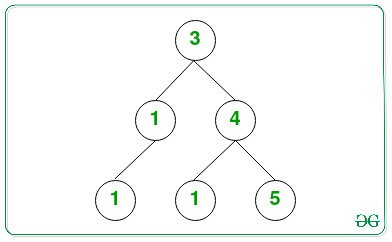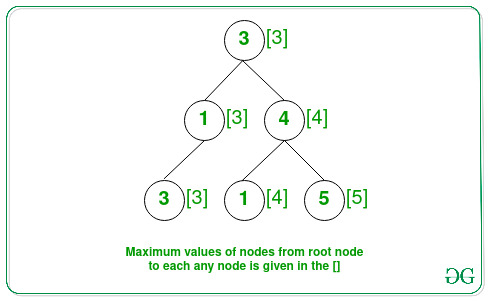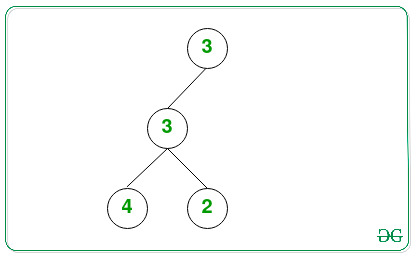# Count paths in a Binary Tree consisting of nodes in non-decreasing order

• Last Updated : 24 Sep, 2021

Given a Binary Tree consisting of N nodes, the task is to find the number of paths from the root to any node X, such that all the node values in that path are at most X.

Examples:

Input: Below is the given Tree:Output: 4
Explanation:The paths from the root to any node X that have value at most values of node X are:

• Node 3(root node): It always follows the given property.
• Node 4: The path starting from the root to node with value 4 has order (3 → 4), with the maximum value of a node being 4.
• Node 5: The path starting from the root to node with value 5 has order (3 → 4 → 5), with the maximum value of a node being 5.
• Node 3: The path starting from the root to node with value 3 has order (3 → 1 → 3), with the maximum value of a node being 3.

Therefore, the count of required paths is 4.

Input: Below is the given Tree:Output: 3

Approach – using DFS: The idea is to traverse the tree using a Depth First Search traversal while checking if the maximum value from root to any node X is equal to X or not.
Follow the steps below to solve the problem:

• Initialize a variable, say count as 0 to store the count of paths from the root to any node X having all the node values in that path is at most X.
• Traverse the tree recursively using depth-first-search and perform the following steps:
• Every recursive call for DFS Traversal, apart from the parent node, pass the maximum value of the node obtained so far in that path.
• Check if the current node value is greater or equal to the maximum value obtained so far, then increment the value of count by 1 and update the maximum value to the current node value.
• After completing the above steps, print the value of count as the result.

Below is the implementation of the above approach:

## C++

 `// C++ program for the above approach`   `#include ` `using` `namespace` `std;`   `// Node structure of the binary tree` `struct` `Node {` `    ``int` `val;` `    ``Node *left, *right;` `};`   `// Function for creating new node` `struct` `Node* newNode(``int` `data)` `{` `    ``// Allocate memory for new node` `    ``struct` `Node* temp = ``new` `Node();`   `    ``// Assigning data value` `    ``temp->val = data;` `    ``temp->left = NULL;` `    ``temp->right = NULL;`   `    ``// Return the Node` `    ``return` `temp;` `}`   `// Function to perform the DFS Traversal` `// to find the number of paths having` `// root to node X has value at most X` `int` `countNodes(Node* root, ``int` `max)` `{` `    ``// If the root node is NULL` `    ``if` `(!root)` `        ``return` `0;`   `    ``// Check if the current value is` `    ``// greater than the maximum value` `    ``// in path from root to current node` `    ``if` `(root->val >= max)` `        ``return` `1 + countNodes(root->left,` `                              ``root->val)` `               ``+ countNodes(root->right, root->val);`   `    ``// Otherwise` `    ``return` `countNodes(root->left,` `                      ``max)` `           ``+ countNodes(root->right,` `                        ``max);` `}`   `// Driver Code` `int` `main()` `{` `    ``// Given Binary Tree` `    ``Node* root = NULL;` `    ``root = newNode(3);` `    ``root->left = newNode(1);` `    ``root->right = newNode(4);` `    ``root->left->left = newNode(3);` `    ``root->right->left = newNode(1);` `    ``root->right->right = newNode(5);`   `    ``cout << countNodes(root, INT_MIN);`   `    ``return` `0;` `}`

## Java

 `// Java program for the above approach` `import` `java.util.*;`   `// Class containing left and` `// right child of current` `// node and key value` `class` `Node {` ` `  `    ``int` `data;` `    ``Node left, right;` ` `  `    ``public` `Node(``int` `item)` `    ``{` `        ``data = item;` `        ``left = right = ``null``;` `    ``}` `}` ` `    `class` `GFG {` `    `  `    ``// Root of the Binary Tree` `    ``Node root;` ` `  `    ``public` `GFG()` `    ``{` `        ``root = ``null``;` `    ``}` `    `  `    ``// Function to perform the DFS Traversal` `// to find the number of paths having` `// root to node X has value at most X` `static` `int` `countNodes(Node root, ``int` `max)` `{` `  `  `    ``// If the root node is NULL` `    ``if` `(root == ``null``)` `        ``return` `0``;`   `    ``// Check if the current value is` `    ``// greater than the maximum value` `    ``// in path from root to current node` `    ``if` `(root.data >= max)` `        ``return` `1` `+ countNodes(root.left,` `                              ``root.data)` `               ``+ countNodes(root.right, root.data);`   `    ``// Otherwise` `    ``return` `countNodes(root.left,` `                      ``max)` `           ``+ countNodes(root.right,` `                        ``max);` `}`   `  ``// Driver code` `public` `static` `void` `main (String[] args) ` `{` `  `  `      ``GFG tree = ``new` `GFG();` `        ``tree.root = ``new` `Node(``3``);` `        ``tree.root.left = ``new` `Node(``1``);` `        ``tree.root.right = ``new` `Node(``4``);` `        ``tree.root.left.left = ``new` `Node(``3``);` `        ``tree.root.right.left = ``new` `Node(``1``);` `        ``tree.root.right.right = ``new` `Node(``5``);` `      ``System.out.println(countNodes(tree.root, Integer.MIN_VALUE));` `    ``}` `}`   `// This code is contributed by offbeat`

## Python3

 `# Python3 program for the above approach`   `# Node structure of the binary tree` `class` `Node:` `    ``def` `__init__(``self``, x):` `        ``self``.val ``=` `x` `        ``self``.left ``=` `None` `        ``self``.right ``=` `None`   `# Function to perform the DFS Traversal` `# to find the number of paths having` `# root to node X has value at most X` `def` `countNodes(root, ``max``):` `    ``# If the root node is NULL` `    ``if` `(``not` `root):` `        ``return` `0`   `    ``# Check if the current value is` `    ``# greater than the maximum value` `    ``#in path from root to current node` `    ``if` `(root.val >``=` `max``):` `        ``return` `1` `+` `countNodes(root.left,root.val) ``+` `countNodes(root.right, root.val)`   `    ``# Otherwise` `    ``return` `countNodes(root.left, ``max``) ``+` `countNodes(root.right, ``max``)`   `# Driver Code` `if` `__name__ ``=``=` `'__main__'``:` `  `  `    ``# Given Binary Tree` `    ``root ``=` `Node(``3``)` `    ``root.left ``=` `Node(``1``)` `    ``root.right ``=` `Node(``4``)` `    ``root.left.left ``=` `Node(``3``)` `    ``root.right.left ``=` `Node(``1``)` `    ``root.right.right ``=` `Node(``5``)`   `    ``print``(countNodes(root, ``-``10``*``*``19``))`   `# This code is contributed by mohit kumar 29.`

## C#

 `// C# program to count frequencies of array items` `using` `System;`   `// Class containing left and` `// right child of current` `// node and key value` `class` `Node {` ` `  `    ``public` `int` `data;` `    ``public` `Node left, right;` ` `  `    ``public` `Node(``int` `item)` `    ``{` `        ``data = item;` `        ``left = right = ``null``;` `    ``}` `}` ` `  `public` `class` `GFG` `{` `    `  `    ``// Root of the Binary Tree` `    ``Node root; ` `    ``public` `GFG()` `    ``{` `        ``root = ``null``;` `    ``}` `    `  `// Function to perform the DFS Traversal` `// to find the number of paths having` `// root to node X has value at most X` `static` `int` `countNodes(Node root, ``int` `max)` `{` `  `  `    ``// If the root node is NULL` `    ``if` `(root == ``null``)` `        ``return` `0;`   `    ``// Check if the current value is` `    ``// greater than the maximum value` `    ``// in path from root to current node` `    ``if` `(root.data >= max)` `        ``return` `1 + countNodes(root.left,` `                              ``root.data)` `               ``+ countNodes(root.right, root.data);`   `    ``// Otherwise` `    ``return` `countNodes(root.left,` `                      ``max)` `           ``+ countNodes(root.right,` `                        ``max);` `}`   `// Driver code` `public` `static` `void` `Main(String []args)` `{` `        ``GFG tree = ``new` `GFG();` `        ``tree.root = ``new` `Node(3);` `        ``tree.root.left = ``new` `Node(1);` `        ``tree.root.right = ``new` `Node(4);` `        ``tree.root.left.left = ``new` `Node(3);` `        ``tree.root.right.left = ``new` `Node(1);` `        ``tree.root.right.right = ``new` `Node(5);` `        ``Console.WriteLine(countNodes(tree.root, Int32.MinValue));` `    ``}` `}`   `// This code is contributed by jana_sayantan.`

## Javascript

 ``

Output:

`4`

Time Complexity: O(N)
Auxiliary Space: O(N)

Approach using BFS: The idea is to traverse the tree using breadth-first search while checking if the maximum value from root to X is equal to X. Follow the steps below to solve the problem:

• Initialize a variable, say count as 0 to store the count of paths from the root to any node X having all the node values in that path is at most X and a queue Q of pairs to perform the BFS Traversal.
• Push the root node with INT_MIN as the maximum value in the queue.
• Now, until Q is non-empty perform the following:
• Pop the front node from the queue.
• If the front node value is at least the current maximum value obtained so far, then increment the value of count by 1.
• Update the maximum value that occurred so far with the current node value.
• If the left and right nodes exist for the current popped node then push it into the queue Q with the updated maximum value in the above step.
• After completing the above steps, print the value of count as the result.

Below is the implementation of the above approach:

## C++

 `// C++ program for the above approach`   `#include ` `using` `namespace` `std;`   `// Node of the binary tree` `struct` `Node {` `    ``int` `val;` `    ``Node *left, *right;` `};`   `// Function for creating new node` `struct` `Node* newNode(``int` `data)` `{` `    ``// Allocate memory for new node` `    ``struct` `Node* temp = ``new` `Node();` `    ``temp->val = data;` `    ``temp->left = NULL;` `    ``temp->right = NULL;`   `    ``// Return the created node` `    ``return` `temp;` `}`   `// Function to perform the DFS Traversal` `// to find the number of paths having` `// root to node X has value at most X` `int` `countNodes(Node* root)` `{` `    ``// Initialize queue` `    ``queue > q;` `    ``int` `m = INT_MIN;`   `    ``// Push root in queue with the` `    ``// maximum value m` `    ``q.push({ root, m });`   `    ``// Stores the count of good nodes` `    ``int` `count = 0;`   `    ``// Traverse all nodes` `    ``while` `(!q.empty()) {`   `        ``// Store the front node of` `        ``// the queue` `        ``auto` `temp = q.front();` `        ``q.pop();` `        ``Node* node = temp.first;` `        ``int` `num = temp.second;`   `        ``// Check is current node is` `        ``// greater than the maximum` `        ``// value in path from root to` `        ``// the current node` `        ``if` `(node->val >= num)` `            ``count++;`   `        ``// Update the maximum value m` `        ``m = max(node->val, num);`   `        ``// If left child is not null,` `        ``// push it to queue with the` `        ``// maximum value m` `        ``if` `(node->left)` `            ``q.push({ node->left, m });`   `        ``// If right child is not null,` `        ``// push it to queue with the` `        ``// maximum value m` `        ``if` `(node->right)` `            ``q.push({ node->right, m });` `    ``}`   `    ``// Returns the answer` `    ``return` `count;` `}`   `// Driver Code` `int` `main()` `{` `    ``// Construct a Binary Tree` `    ``Node* root = NULL;` `    ``root = newNode(3);` `    ``root->left = newNode(1);` `    ``root->right = newNode(4);` `    ``root->left->left = newNode(3);` `    ``root->right->left = newNode(1);` `    ``root->right->right = newNode(5);`   `    ``cout << countNodes(root);`   `    ``return` `0;` `}`

## Java

 `// Java program for the above approach` `import` `java.util.*;`   `// Node of the binary tree` `class` `Node {` `  `  `    ``int` `val;` `    ``Node left, right;` `  `  `    ``public` `Node(``int` `data)` `    ``{` `        ``val = data;` `        ``left = right = ``null``;` `    ``}` `}`   `// User defined Pair class` `class` `Pair {` `    ``Node x;` `    ``int` `y;` `  `  `    ``// Constructor` `    ``public` `Pair(Node x, ``int` `y)` `    ``{` `        ``this``.x = x;` `        ``this``.y = y;` `    ``}` `}` `    `  `public` `class` `Main` `{` `    ``// Function for creating new node` `    ``static` `Node newNode(``int` `data)` `    ``{` `        ``// Allocate memory for new node` `        ``Node temp = ``new` `Node(data);` `      `  `        ``// Return the created node` `        ``return` `temp;` `    ``}` `      `  `    ``// Function to perform the DFS Traversal` `    ``// to find the number of paths having` `    ``// root to node X has value at most X` `    ``static` `int` `countNodes(Node root)` `    ``{` `        ``// Initialize queue` `        ``Vector q = ``new` `Vector();` `        ``int` `m = Integer.MIN_VALUE;` `      `  `        ``// Push root in queue with the` `        ``// maximum value m` `        ``q.add(``new` `Pair(root, m));` `      `  `        ``// Stores the count of good nodes` `        ``int` `count = ``0``;` `      `  `        ``// Traverse all nodes` `        ``while` `(q.size() > ``0``) {` `      `  `            ``// Store the front node of` `            ``// the queue` `            ``Pair temp = q.get(``0``);` `            ``q.remove(``0``);` `            ``Node node = temp.x;` `            ``int` `num = temp.y;` `      `  `            ``// Check is current node is` `            ``// greater than the maximum` `            ``// value in path from root to` `            ``// the current node` `            ``if` `(node.val >= num)` `                ``count++;` `      `  `            ``// Update the maximum value m` `            ``m = Math.max(node.val, num);` `      `  `            ``// If left child is not null,` `            ``// push it to queue with the` `            ``// maximum value m` `            ``if` `(node.left != ``null``)` `                ``q.add(``new` `Pair(node.left, m));` `      `  `            ``// If right child is not null,` `            ``// push it to queue with the` `            ``// maximum value m` `            ``if` `(node.right != ``null``)` `                ``q.add(``new` `Pair(node.right, m));` `        ``}` `      `  `        ``// Returns the answer` `        ``return` `count;` `    ``}`   `    ``public` `static` `void` `main(String[] args) {` `        ``// Construct a Binary Tree` `        ``Node root = ``null``;` `        ``root = newNode(``3``);` `        ``root.left = newNode(``1``);` `        ``root.right = newNode(``4``);` `        ``root.left.left = newNode(``3``);` `        ``root.right.left = newNode(``1``);` `        ``root.right.right = newNode(``5``);` `      `  `        ``System.out.println(countNodes(root));` `    ``}` `}`   `// This code is contributed by mukesh07.`

## Python3

 `# Python3 program for the above approach` `import` `sys `   `# Node of the binary tree` `class` `Node:` `    ``def` `__init__(``self``, data):` `        ``self``.val ``=` `data` `        ``self``.left ``=` `None` `        ``self``.right ``=` `None`   `# Function for creating new node` `def` `newNode(data):` `    ``# Allocate memory for new node` `    ``temp ``=` `Node(data)`   `    ``# Return the created node` `    ``return` `temp`   `# Function to perform the DFS Traversal` `# to find the number of paths having` `# root to node X has value at most X` `def` `countNodes(root):` `    ``# Initialize queue` `    ``q ``=` `[]` `    ``m ``=` `-``sys.maxsize`   `    ``# Push root in queue with the` `    ``# maximum value m` `    ``q.append([ root, m ])`   `    ``# Stores the count of good nodes` `    ``count ``=` `0`   `    ``# Traverse all nodes` `    ``while` `(``len``(q) > ``0``):` `        ``# Store the front node of` `        ``# the queue` `        ``temp ``=` `q[``0``]` `        ``q.pop(``0``)` `        ``node ``=` `temp[``0``]` `        ``num ``=` `temp[``1``]`   `        ``# Check is current node is` `        ``# greater than the maximum` `        ``# value in path from root to` `        ``# the current node` `        ``if` `(node.val >``=` `num):` `            ``count``+``=``1`   `        ``# Update the maximum value m` `        ``m ``=` `max``(node.val, num)`   `        ``# If left child is not null,` `        ``# push it to queue with the` `        ``# maximum value m` `        ``if` `(node.left !``=` `None``):` `            ``q.append([ node.left, m ])`   `        ``# If right child is not null,` `        ``# push it to queue with the` `        ``# maximum value m` `        ``if` `(node.right !``=` `None``):` `            ``q.append([ node.right, m ])`   `    ``# Returns the answer` `    ``return` `count`   `# Construct a Binary Tree` `root ``=` `None` `root ``=` `newNode(``3``)` `root.left ``=` `newNode(``1``)` `root.right ``=` `newNode(``4``)` `root.left.left ``=` `newNode(``3``)` `root.right.left ``=` `newNode(``1``)` `root.right.right ``=` `newNode(``5``)`   `print``(countNodes(root))`   `# This code is contributed by rameshtravel07.`

## C#

 `// C# program for the above approach` `using` `System;` `using` `System.Collections;` `class` `GFG {` `    `  `    ``// Node of the binary tree` `    ``class` `Node {` `       `  `        ``public` `int` `val;` `        ``public` `Node left, right;` `       `  `        ``public` `Node(``int` `data)` `        ``{` `            ``val = data;` `            ``left = right = ``null``;` `        ``}` `    ``}` `    `  `    ``// Function for creating new node` `    ``static` `Node newNode(``int` `data)` `    ``{` `        ``// Allocate memory for new node` `        ``Node temp = ``new` `Node(data);` `     `  `        ``// Return the created node` `        ``return` `temp;` `    ``}` `     `  `    ``// Function to perform the DFS Traversal` `    ``// to find the number of paths having` `    ``// root to node X has value at most X` `    ``static` `int` `countNodes(Node root)` `    ``{` `        ``// Initialize queue` `        ``Queue q = ``new` `Queue();` `        ``int` `m = Int32.MinValue;` `     `  `        ``// Push root in queue with the` `        ``// maximum value m` `        ``q.Enqueue(``new` `Tuple(root, m));` `     `  `        ``// Stores the count of good nodes` `        ``int` `count = 0;` `     `  `        ``// Traverse all nodes` `        ``while` `(q.Count > 0) {` `     `  `            ``// Store the front node of` `            ``// the queue` `            ``Tuple temp = (Tuple)q.Peek();` `            ``q.Dequeue();` `            ``Node node = temp.Item1;` `            ``int` `num = temp.Item2;` `     `  `            ``// Check is current node is` `            ``// greater than the maximum` `            ``// value in path from root to` `            ``// the current node` `            ``if` `(node.val >= num)` `                ``count++;` `     `  `            ``// Update the maximum value m` `            ``m = Math.Max(node.val, num);` `     `  `            ``// If left child is not null,` `            ``// push it to queue with the` `            ``// maximum value m` `            ``if` `(node.left != ``null``)` `                ``q.Enqueue(``new` `Tuple(node.left, m));` `     `  `            ``// If right child is not null,` `            ``// push it to queue with the` `            ``// maximum value m` `            ``if` `(node.right != ``null``)` `                ``q.Enqueue(``new` `Tuple(node.right, m));` `        ``}` `     `  `        ``// Returns the answer` `        ``return` `count;` `    ``}` `    `  `  ``// Driver code` `  ``static` `void` `Main()` `  ``{` `    `  `    ``// Construct a Binary Tree` `    ``Node root = ``null``;` `    ``root = newNode(3);` `    ``root.left = newNode(1);` `    ``root.right = newNode(4);` `    ``root.left.left = newNode(3);` `    ``root.right.left = newNode(1);` `    ``root.right.right = newNode(5);` ` `  `    ``Console.Write(countNodes(root));` `  ``}` `}`   `// This code is contributed by decode2207.`

## Javascript

 ``

Output:

`4`

Time Complexity: O(N)
Auxiliary Space: O(N)

My Personal Notes arrow_drop_up
Recommended Articles
Page :